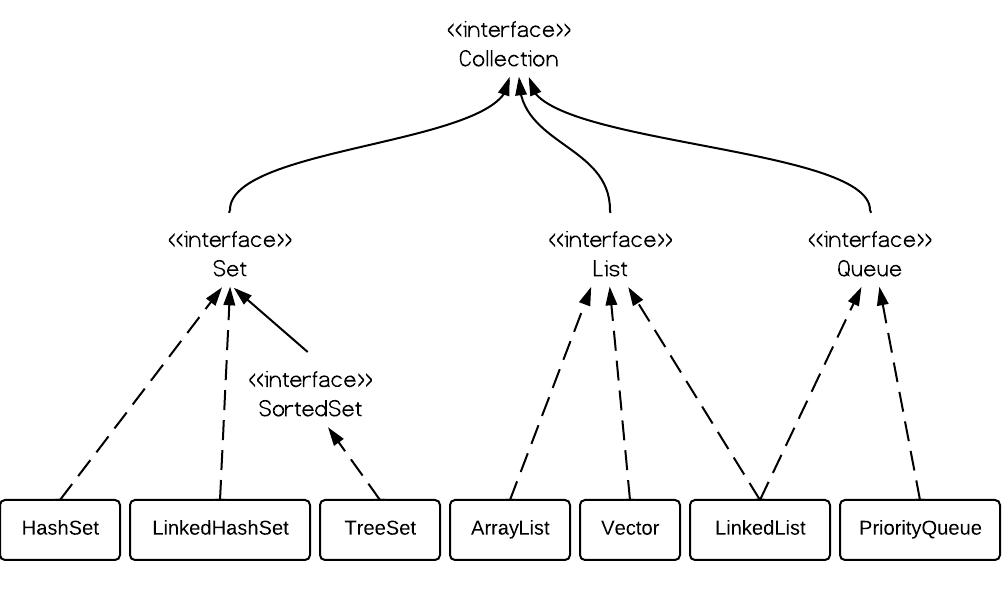# ArrayList vs. LinkedList vs. Vector

· Java Zone · Analysis
Save
581.08K Views

### 1. list overview

list, as its name indicates, is an ordered sequence of elements. when we talk about list, it is a good idea to compare it with set which is a set of elements which is unordered and every element is unique.  the following is the class hierarchy diagram of collection.### 2. arraylist vs. linkedlist vs. vector

from the hierarchy diagram, they all implement list interface. they are very similar to use. their main difference is their implementation which causes different performance for different operations.  arraylist is implemented as a resizable array. as more elements are added to arraylist, its size is increased dynamically. it's elements can be accessed directly by using the get and set methods, since arraylist is essentially an array. linkedlist is implemented as a double linked list. its performance on add and remove is better than arraylist, but worse on get and set methods. vector is similar with arraylist, but it is synchronized. arraylist is a better choice if your program is thread-safe. vector and arraylist require space as more elements are added. vector each time doubles its array size, while arraylist grow 50% of its size each time. linkedlist, however, also implements queue interface which adds more methods than arraylist and vector, such as offer(), peek(), poll(), etc.    note: the default initial capacity of an arraylist is pretty small. it is a good habit to construct the arraylist with a higher initial capacity. this can avoid the resizing cost.

### 3. arraylist example

``````arraylist al = new arraylist();
al.add(3);
al.add(2);
al.add(1);
al.add(4);
al.add(5);
al.add(6);
al.add(6);

iterator iter1 = al.iterator();
while(iter1.hasnext()){
system.out.println(iter1.next());
}
``````

### 4. linkedlist example

``````linkedlist ll = new linkedlist();
ll.add(3);
ll.add(2);
ll.add(1);
ll.add(4);
ll.add(5);
ll.add(6);
ll.add(6);

iterator iter2 = al.iterator();
while(iter2.hasnext()){
system.out.println(iter2.next());
}
``````

as shown in the examples above, they are similar to use. the real difference is their underlying implementation and their operation complexity.

5. vector

vector is almost identical to arraylist, and the difference is that vector is synchronized. because of this, it has an overhead than arraylist. normally, most java programmers use arraylist instead of vector because they can synchronize explicitly by themselves.

### 6. performance of arraylist vs. linkedlist

the time complexity comparison is as follows:

i use the following code to test their performance:
``````arraylist arraylist = new arraylist();
linkedlist linkedlist = new linkedlist();

// arraylist add
long starttime = system.nanotime();

for (int i = 0; i < 100000; i++) {
arraylist.add(i);
}
long endtime = system.nanotime();
long duration = endtime - starttime;
system.out.println("arraylist add:  " + duration);

// linkedlist add
starttime = system.nanotime();

for (int i = 0; i < 100000; i++) {
linkedlist.add(i);
}
endtime = system.nanotime();
duration = endtime - starttime;
system.out.println("linkedlist add: " + duration);

// arraylist get
starttime = system.nanotime();

for (int i = 0; i < 10000; i++) {
arraylist.get(i);
}
endtime = system.nanotime();
duration = endtime - starttime;
system.out.println("arraylist get:  " + duration);

// linkedlist get
starttime = system.nanotime();

for (int i = 0; i < 10000; i++) {
linkedlist.get(i);
}
endtime = system.nanotime();
duration = endtime - starttime;
system.out.println("linkedlist get: " + duration);

// arraylist remove
starttime = system.nanotime();

for (int i = 9999; i >=0; i--) {
arraylist.remove(i);
}
endtime = system.nanotime();
duration = endtime - starttime;
system.out.println("arraylist remove:  " + duration);

// linkedlist remove
starttime = system.nanotime();

for (int i = 9999; i >=0; i--) {
linkedlist.remove(i);
}
endtime = system.nanotime();
duration = endtime - starttime;
system.out.println("linkedlist remove: " + duration);
``````
and the output is:
``````arraylist add: 13265642
linkedlist add: 9550057
arraylist get: 1543352
linkedlist get: 85085551
arraylist remove: 199961301
linkedlist remove: 85768810``````

the difference of their performance is obvious. linkedlist is faster in add and remove, but slower in get. based on the complexity table and testing results, we can figure out when to use arraylist or linkedlist.
in brief, linkedlist should be preferred if:
• there are no large number of random access of element
• there are a large number of add/remove operations
Topics:
java, arraylist, linkedlist, vector

Published at DZone with permission of Ryan Wang.

Opinions expressed by DZone contributors are their own.

Comments